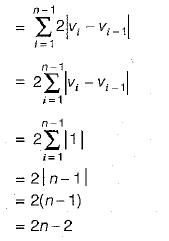Courses

# Greedy Method (Basic Level) - 1

## 10 Questions MCQ Test Question Bank for GATE Computer Science Engineering | Greedy Method (Basic Level) - 1

Description
This mock test of Greedy Method (Basic Level) - 1 for Computer Science Engineering (CSE) helps you for every Computer Science Engineering (CSE) entrance exam. This contains 10 Multiple Choice Questions for Computer Science Engineering (CSE) Greedy Method (Basic Level) - 1 (mcq) to study with solutions a complete question bank. The solved questions answers in this Greedy Method (Basic Level) - 1 quiz give you a good mix of easy questions and tough questions. Computer Science Engineering (CSE) students definitely take this Greedy Method (Basic Level) - 1 exercise for a better result in the exam. You can find other Greedy Method (Basic Level) - 1 extra questions, long questions & short questions for Computer Science Engineering (CSE) on EduRev as well by searching above.
QUESTION: 1

### 6 files F1, F2, F3, F4, F5and F6 have 100, 200, 50, 80, 120, 150 records respectively. In what order should they be stored so as to optimize act. Assume each file is accessed with the same frequency.

Solution:

Since the access is sequential, greater the distance, greater will be the access time. Since all the files are referenced with equal frequency, overall access time can be reduced by arranging them as then ascending order of record as in option (a).

QUESTION: 2

### Which of the following algorithms solves the all pair shortest path problem?

Solution:

Dijkstra’s algorithm solves single source shortest path problem.
Warshall’s algorithm finds transitive closure of a given graph.
Prim’s algorithm constructs a minimum cost spanning tree for a given weighted graph.

QUESTION: 3

### Consider the graph in figure:​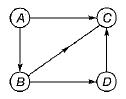Which of the following is a valid topological sorting?

Solution:

In topological sorting we have to list out all the nodes in such a way that whenever there is an edge connecting i and j, i should precede j in the listing, For some graphs, this is not at all possible, for some this can be done in more than one way.

QUESTION: 4

Kruskal’s algorithm for finding a minimum spanning tree of a weighted graph G with n vertices and m edges has the time complexity of

Solution:

Kruskal’s algorithm time complexity
= O(e log v)
= O(m log n)

QUESTION: 5

Let G be an undirected connected graph with distinct edge weights. Let emax be the edge with maximum weight and emin the edge with minimum weight. Which of the following statements is false?

Solution:

All edges are distinct weights.
Removal of emax may not disconnect G, because other edges may cover the vertices incident with emax.

QUESTION: 6

Consider the undirected graph below: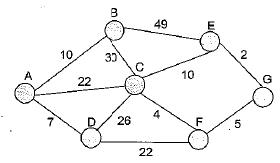Using Prim’s algorithm to construct a minimum spanning tree starting with node A, which one of the following sequences of edges represents a possible order in which the edges would be added to construct the minimum spanning tree?

Solution:

Prim’s algorithm having special property which is, while finding minimum cost spanning tree, graph always be connected.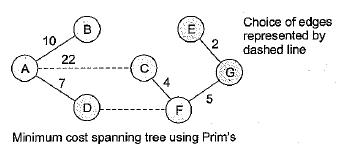Now for correct options, we need to check while entry for any edge except for 1st edge only one vertex match from all left side (i.e. visited edges) edges.
(a) (E, G), (C, F ) : here for edge (C, F) no vertex match to (E, G). So not possible using Prim’s.
(b) (A, D), (A, B), (A, C), (C, F), (G, E ) : here for edge (G, E) no vertex match to any edge present left side of it so not possible using Prim’s.
(c) (A, B), (A, D), (D, F), (F, G), (G, E), (F, C) not violated rule i.e. always connected in construction of MST.
So True.

QUESTION: 7

In the following table, the left column contains the names of standard graph algorithms and the right column contains the time complexities of the algorithms. Match each algorithm with its time complexity.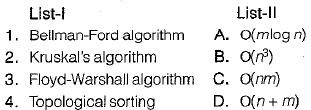Solution:

Bellman-Ford algorithm: O{nm)
Kruskal’s algorithm : O(mlog n)
Floyd-Warshall algorithm: O(n3)

Topological sorting : O(n + m)

QUESTION: 8

Let G{V, E) an undirected graph with positive edge weights. Dijkstra’s single source-shortest path algorithmn can be implemented using the binary heap data structure with time complexity?

Solution:

Dijkstra’s implementation for single source shortest path

(i) Using binary heap takes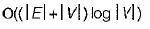(ii) .Using Fibonacci heap takes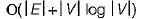QUESTION: 9

Consider the polynominal p(x) = a0 + a1x + a2x2 + a3x3, where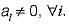The minimum number of multiplications needed to evaluate p on an input x is

Solution: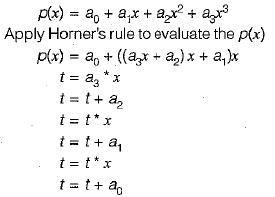So, 3 minimum number of multiplications needed to evaluate p on an input x.

QUESTION: 10

Consider a weighted complete graph G on the vertex set {v1, v2, .... vn} such that the weight of the edge (vi, vj) is 2 | i - j | .The weight of a minimum

Solution: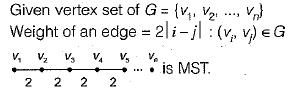In the case of minimum spanning tree of a graph G we will add up to ( n - 1) vertices, so the weight of a minimum spanning tree of G